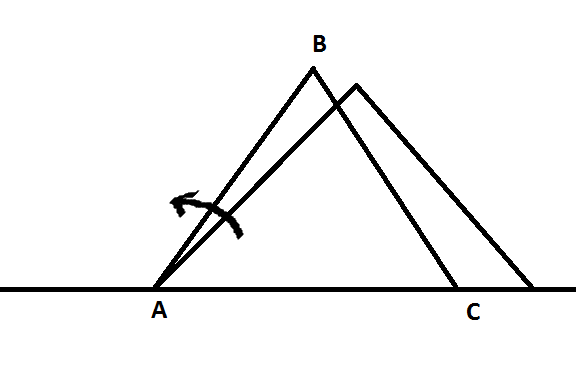# Constrained Motion (JEE)One end $A$ of rod $AB$ (length $4 \ \text{m}$) is pivoted to the ground, while the other end $B$ is pivoted to the rod $BC$ (also length $4 \ \text{m}$). The end $C$ of rod $BC$ is such that it can only slide on the ground in a straight line. It cannot be lifted.

If the rod $AB$ rotates about $A$ with $\omega=1 \ \text{rad/s}$ as shown, then find the speed, in m/s, of the end $C$ when $\angle ABC=60^{o}$.

×

Problem Loading...

Note Loading...

Set Loading...